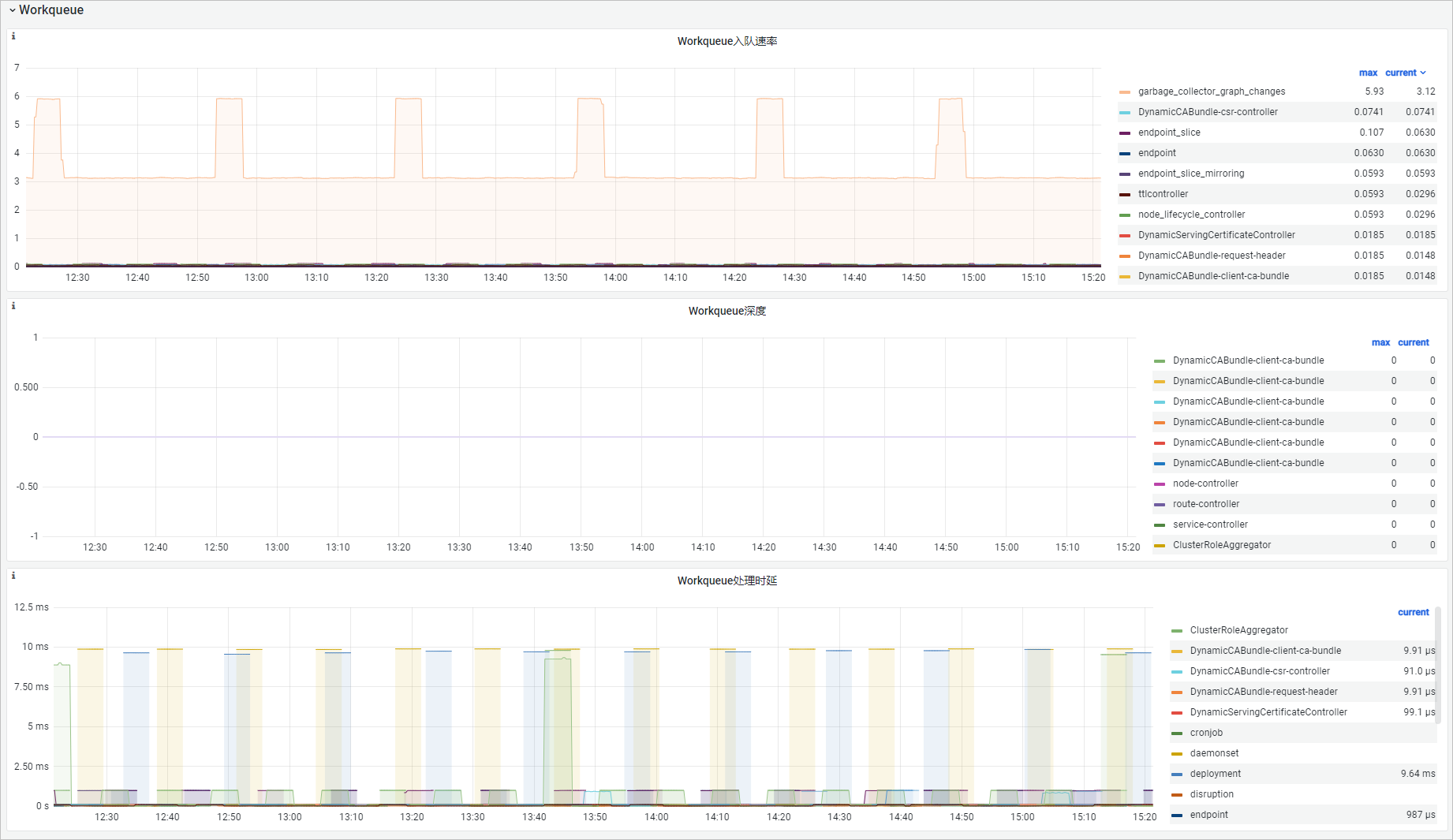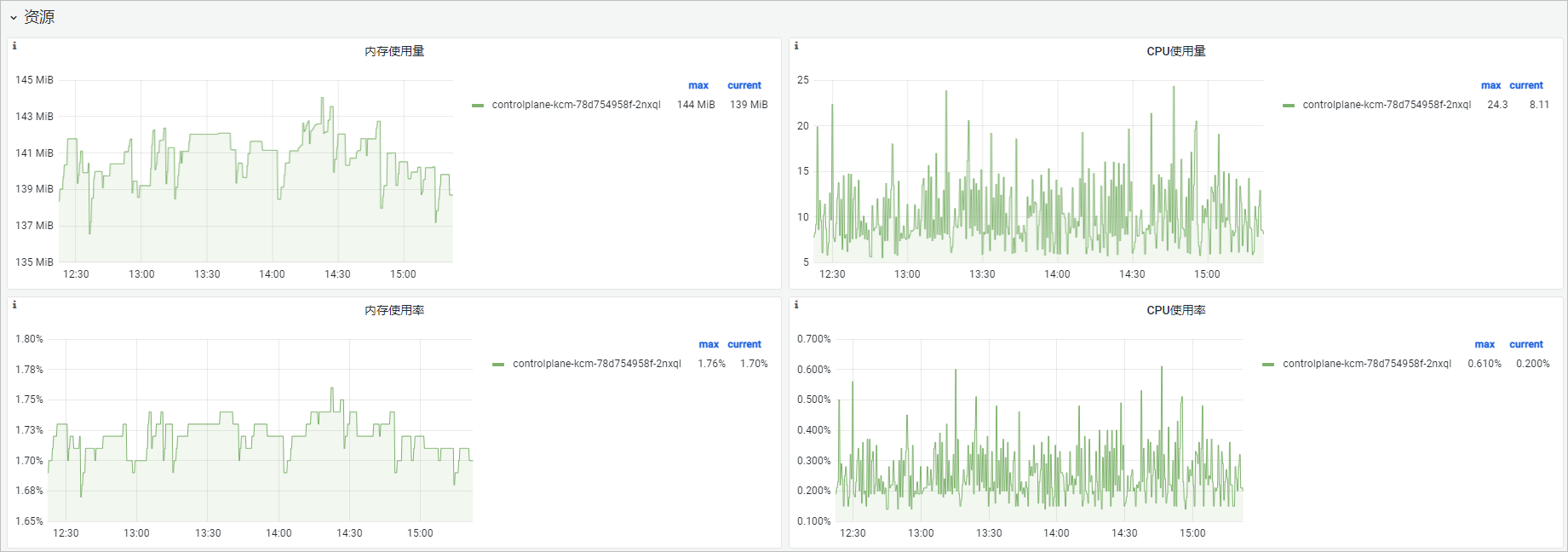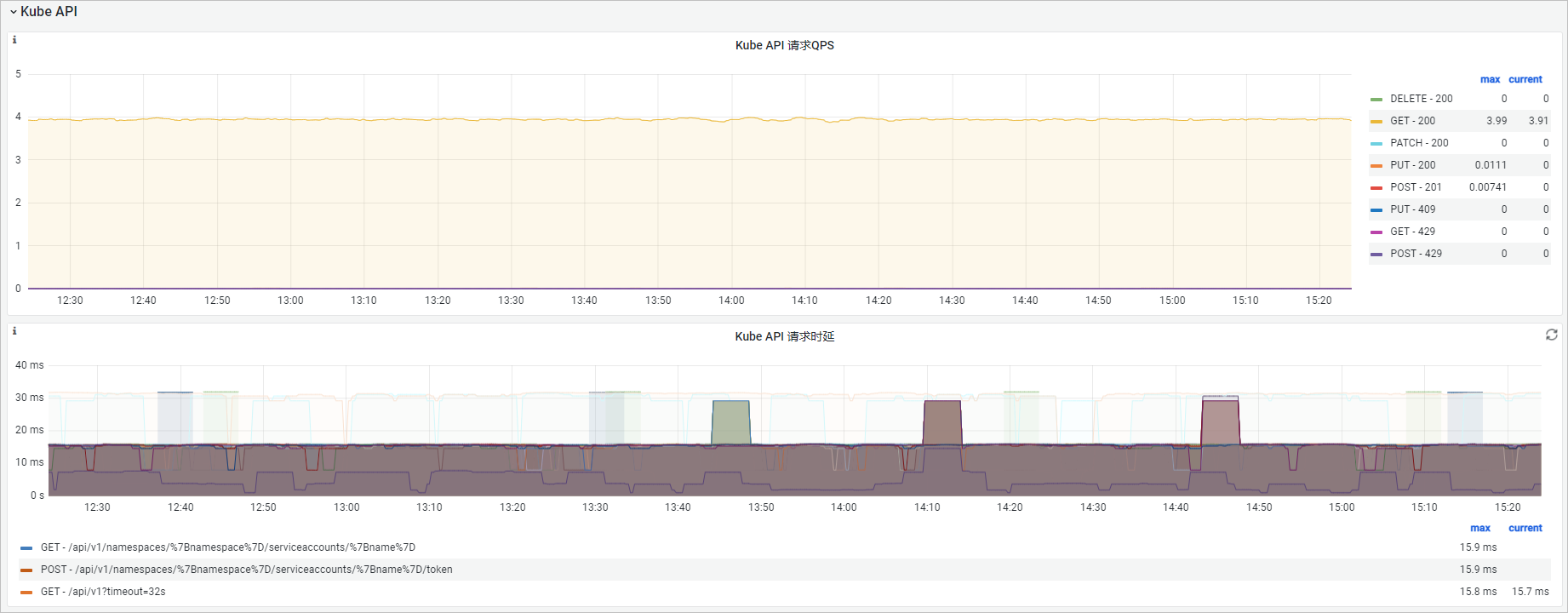## 指标清单

workqueue_depthGaugeWorkqueue当前队列深度。
workqueue_queue_duration_seconds_bucketHistogram任务在Workqueue中存在的时长。
memory_utilization_byteGauge内存使用量，单位：字节（Byte）。
memory_utilization_ratioGauge内存使用率=内存使用量/内存资源上限，百分比形式。
cpu_utilization_coreGaugeCPU使用量，单位：核（Core）。
cpu_utilization_ratioGaugeCPU使用率=CPU使用量/内存资源上限，百分比形式。
rest_client_requests_totalCounter从状态值（Status Code）、方法（Method）和主机（Host）维度分析HTTP请求数。
rest_client_request_duration_seconds_bucketHistogram从方法（Verb）和URL维度分析HTTP请求时延。

## 大盘使用指导

### Workqueue

• 可观测性展示• 功能解析
大盘名称PromQL说明
Workqueue深度sum(rate(workqueue_depth{job="ack-kube-controller-manager"}[\$interval])) by (name)
Workqueue处理时延histogram_quantile(\$quantile, sum(rate(workqueue_queue_duration_seconds_bucket{job="ack-kube-controller-manager"}[5m])) by (name, le))

### 资源

• 可观测性展示• 功能解析

CPU使用量cpu_utilization_core{container="kube-controller-manager"}*1000CPU使用量，单位：毫核。

CPU使用率cpu_utilization_ratio{container="kube-controller-manager"}CPU使用率，百分比。

### Kube API

• 可观测性展示• 功能解析
大盘名称PromQL说明
Kube API请求QPS
• sum(rate(rest_client_requests_total{job="ack-scheduler",code=~"2.."}[\$interval])) by (method,code)
• sum(rate(rest_client_requests_total{job="ack-scheduler",code=~"3.."}[\$interval])) by (method,code)
• sum(rate(rest_client_requests_total{job="ack-scheduler",code=~"4.."}[\$interval])) by (method,code)
• sum(rate(rest_client_requests_total{job="ack-scheduler",code=~"5.."}[\$interval])) by (method,code)
对kube-apiserver发起的HTTP请求，从方法（Method）和返回值（Code) 维度分析。
Kube API请求时延histogram_quantile(\$quantile, sum(rate(rest_client_request_duration_seconds_bucket{job="ack-kube-controller-manager"}[\$interval])) by (verb,url,le))对kube-apiserver发起的HTTP请求时延，从方法（Verb）和请求URL维度分析。